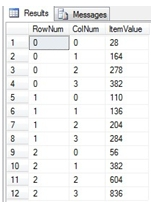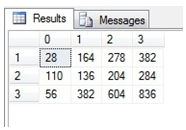# SQL Server MMULT function

MMULT

Updated: 17 August 2010

Use MMULT to calculate the matrix product of two arrays. The result is a TABLE where the maximum row number is equal to the number of rows in the first array and the maximum column number is the number of columns in the second array

For matrices in normalized form, use the MMULTN function.
Syntax
SELECT * FROM [wctMath].[wct].[MMULT] (
<@Matrix_A_TableName, nvarchar(4000),>
,<@Matrix_A_ColumnNames, nvarchar(4000),>
,<@Matrix_A_GroupedColumnName, nvarchar(4000),>
,<@Matrix_A_GroupedColumnValue, sql_variant,>
,<@Matrix_B_TableName, nvarchar(4000),>
,<@Matrix_B_ColumnNames, nvarchar(4000),>
,<@Matrix_B_GroupedColumnName, nvarchar(4000),>
,<@Matrix_B_GroupedColumnValue, sql_variant,>)
Arguments
@Matrix_A_TableName
the name, as text, of the table or view that contains the values in the first array to be used in the MMULT calculation.
@Matrix_A_ColumnNames
the name, as text, of the columns in the table or view specified by @Matrix_A_TableName that contains the array values to be used in the MMULT calculation. Data returned from the @Matrix_A_ColumnNames must be of the type float or of a type that implicitly converts to float.
@Matrix_A_GroupedColumnName
the name, as text, of the column in the table or view specified by @Matrix_A_TableName which will be used for grouping the results.
@Matrix_A_GroupedColumnValue
the column value to do the grouping on.
@Matrix_B_TableName
the name, as text, of the table or view that contains the values in the second array to be used in the MMULT calculation.
@Matrix_B_ColumnNames
the name, as text, of the columns in the table or view specified by @Matrix_B_TableName that contains the array values to be used in the MMULT calculation. Data returned from the @Matrix_B_ColumnNames must be of the type float or of a type that implicitly converts to float.
@Matrix_B_GroupedColumnName
the name, as text, of the column in the table or view specified by @Matrix_B_TableName which will be used for grouping the results.
@Matrix_B_GroupedColumnValue
the column value to do the grouping on.
Return Types
TABLE (
[RowNum] [int] NULL,
[ColNum] [int] NULL,
[ItemValue] [float] NULL
Remarks
·         The number of columns in the ‘A’ array must be equal to the number of rows in the ‘B’ array or an error will be returned.
·         Use the MMULT_q function for more complex queries or to pass the matrices directly into the function without having to store them in a table.
·         Use MMULTN for a table in normal form.
·         If the array contains NULL, then NULL will be returned.
·         If the array contains a blank, it will be treated as zero.
·         The function returns an error if either array contains a non-numeric value.
Examples
In this example, we have created a simple table to store matrices that might have up to 10 columns in them. In practice, the 4,000-character length of @Matrix_A_ColumnNames and @Matrix_B_ColumnNames limits the number of columns that can be handled by MMULT. If you need more than 4,000 characters, use the MMULT_q function
CREATE TABLE #m (
MatrixID      nvarchar(5),
rowno         float,
Col00         float,
Col01         float,
Col02         float,
Col03         float,
Col04         float,
Col05         float,
Col06         float,
Col07         float,
Col08         float,
Col09         float
)

INSERT INTO #m (Matrixid, rowno, Col00, col01, col02, col03) VALUES ('1A',0,5,6,7,8)
INSERT INTO #m (Matrixid, rowno, Col00, col01, col02, col03) VALUES ('1A',1,9,10,-11,12)
INSERT INTO #m (Matrixid, rowno, Col00, col01, col02, col03) VALUES ('1A',2,16,15,14,13)
INSERT INTO #m (Matrixid, rowno, Col00, col01, col02, col03) VALUES ('1B',0,1,8,9,13)
INSERT INTO #m (Matrixid, rowno, Col00, col01, col02, col03) VALUES ('1B',1,2,7,10,14)
INSERT INTO #m (Matrixid, rowno, Col00, col01, col02, col03) VALUES ('1B',2,-3,6,11,15)
INSERT INTO #m (Matrixid, rowno, Col00, col01, col02, col03) VALUES ('1B',3,4,5,12,16)

SELECT C.*
FROM wct.MMULT('#m'
,'col00, col01, col02, col03'
,'MatrixID'
,'1A'
,'#m'
,'col00, col01, col02, col03'
,'MatrixID'
,'1B'
) C

This produces the following resultIf we wanted to return the results in matrix form, we can use the PIVOT function, though this requires knowing the number of columns returned by the function (which is the number of columns in the second array).

SELECT ,,,
FROM (
SELECT C.*
FROM wct.MMULT('#m'
,'col00, col01, col02, col03'
,'MatrixID'
,'1A'
,'#m'
,'col00, col01, col02, col03'
,'MatrixID'
,'1B'
) C
) M PIVOT (
MAX(ItemValue)
FOR colnum IN (,,,)
) AS pvt
ORDER BY rownum

This produces the following resultCopyright 2008-2021 Westclintech LLC         Privacy Policy        Terms of Service